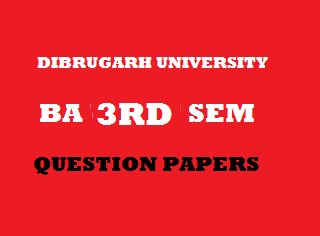## Wednesday, January 02, 20192012
(November)
MATHEMATICS
(General)
Course: 301
[Group A: Coordinate Geometry and
Group B: Analysis –I (Real Analysis)]
Full Marks: 80
Pass Marks: 32
Time: 3 hours
The figures in the margin indicate full marks for the questions
GROUP – A
(Coordinate Geometry)
1. (a) Find where the origin is to be shifted without changing the direction of the axes, so that the terms in x and y are removed from the equationOr
If any angular displacement of the coordinate axes transforms the expressioninto the expressionthen show that2. (a) Write the condition that the straight lines represented byare perpendicular to each other.
(b) Show that a homogeneous equation of the second degree always represents a pair of straight lines passing through the origin. 3
(c) Find the equations of the pair of straight lines represented by 3Or
If the pairs of straight linesandbe such that each pair bisects the angle between the other pair, then prove that(d) If the equationrepresents a pair of straight lines equidistant from the origin, show thatOr
Show that the area of the triangle formed by the lines represented byandis3. (a)
(b)
(c)
Or
Find the condition that the linesbe conjugate diameters of the conicSECTION – II
(3-Dimension)

4. (a) Reduce the equation of the planeto the normal form.
(b) Write the equation of the plane parallel and at unit distance toplane.
(c) Express the equation of thein symmetrical form.
(d) Find the equation of the plane through the pointsandand perpendicular to the plane. 3
Or
Find the equation of the plane passing through the intersection of the planesand perpendicular to the plane.
(e) Find the equation of the two planes represented by the equation 3+1=4Find the angle between them.
Or
Find the coordinates of the foot of the perpendicular drawn from the pointto the plane. Find also the image of the point with respect to the plane.
5. (a) Find the shortest distance between the lines 3andand show that they are coplanar.
Or
A perpendicular is drawn from the origin to the lineFind the equation of the perpendicular and coordinates of its foot.
(b) Find the equation of the line of the shortest distance between the lines 4andOr
Find the surface generated by a straight line which meets the two linesat the same angle.
GROUP – B
(Analysis – I)

6. (a) Find thederivative of, whereand.
(b) Find the curve whose curvature at any point on it is zero.
(c) Prove that the subnormal at any point of a parabola is of constant length.
(d) Evaluate:Or
If, show that7. (a) Verify Roll’s theorem for the functionin2
(b) Write the remainder afterterm of Taylor’s series in Lagrange’s Form. 1
(c) State and prove Lagrange’s mean value theorem. 4
Or
Using Maclaurin’s theorem, expand sin x in an infinite series in powers of x.
(d) State Darboux’s theorem.
(e) Show that functiondefined byIs continuous at8. (a) If, then show that(b) If, prove that. 2
9. (a) Prove that, if 3Or
Show that(b) If,being a positive integer greater than 1, then deduces that, hence find the value of.
Or
Find the whole length of the curve.
(c) Show that,, m, n being positive integers greater than one, then show that.
Or
Using reduction formula ofDeduce***